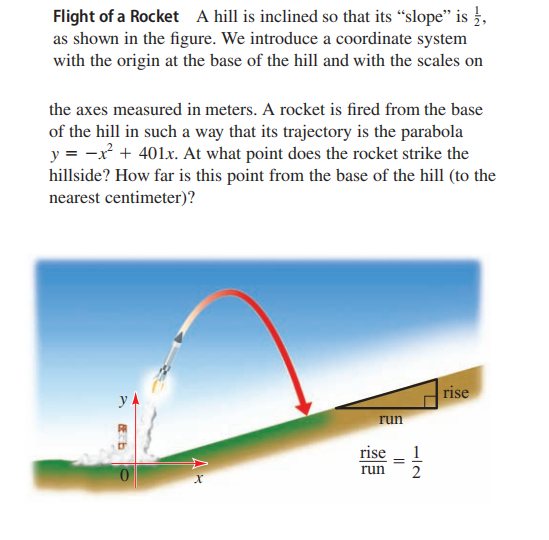### ¿Todavía tienes preguntas de matemáticas?

Pregunte a nuestros tutores expertos
Algebra
PreguntaA hill is inclined so that its "slope" is $$\frac { 1 } { 2 }$$ , as shown in the figure. We introduce a coordinate system with the origin at the base of the hill and with the scales on the axes measured in meters. A rocket is fired from the base of the hill in such a way that its trajectory is the parabola $$y = - x ^ { 2 } + 401 x$$ . At what point does the rocket strike the hillside? How far is this point from the base of the hill (to the nearest centimeter)?

$$( 400.50,200.25 ) , 447.77 m$$